Electron. J. Differential Equations, Vol. 2019 (2019), No. 94, pp. 1-17.

### Linearized stability implies asymptotic stability for radially symmetric equilibria of p-Laplacian boundary value problems in the unit ball in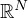Bryan P. Rynne

Abstract:
We consider the parabolic initial-boundary value problem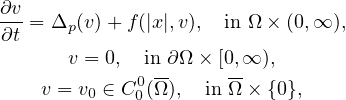where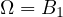is the unit ball centered at the origin in, with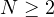,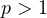, and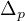denotes the p-Laplacian on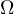. The function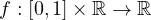is continuous, and the partial derivative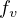exists and is continuous and bounded on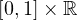. It will be shown that (under certain additional hypotheses) the "principle of linearized stability" holds for radially symmetric equilibrium solutions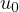of the equation. That is, the asymptotic stability, or instability, ofis determined by the sign of the principal eigenvalue of a linearization of the problem at. It is well-known that this principle holds for the semilinear case p=2 (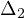is the linear Laplacian), but has not been shown to hold when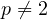. We also consider a bifurcation type problem similar to the one above, having a line of trivial solutions and a curve of non-trivial solutions bifurcating from the line of trivial solutions at the principal eigenvalue of the p-Laplacian. We characterize the stability, or instability, of both the trivial solutions and the non-trivial bifurcating solutions, in a neighbourhood of the bifurcation point, and we obtain a result on "exchange of stability" at the bifurcation point, analogous to the well-known result when p=2.

Submitted November 27, 2018. Published July 29, 2019.
Math Subject Classifications: 35B35, 35B32, 35K55.
Key Words: p-Laplacian; parabolic boundary value problem; stability; bifurcation.

Show me the PDF file (384 KB), TEX file for this article.Bryan P. Rynne Department of Mathematics and the Maxwell Institute for Mathematical Sciences Heriot-Watt University Edinburgh EH14 4AS, Scotland, UK email: B.P.Rynne@hw.ac.uk